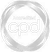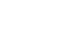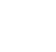# Effective Interest Rates and Discount Factors25 years: Treasury & ALM

In this second part of Moorad's introduction to the time value of money, he explains effective interest rates, present value and discount factors.

Financial MathsIn this second part of Moorad's introduction to the time value of money, he explains effective interest rates, present value and discount factors.

Finance Unlocked is the video learning platform built for finance professionals.### Effective Interest Rates and Discount Factors

9 mins 58 secs

Overview

This further explanation of the time value of money includes calculations of effective rates, present value and the use of discount factors.

Key learning objectives:

• Understand the need and use of effective interest rates

• Use discount factors to calculate the present value

## Book a demo to access

Summary

#### What are effective interest rates?

The interest rate quoted on a deposit or loan is often the flat rate. However, we are often required to compare two interest rates which apply for a similar investment period but have different interest payment frequencies, for example, a two-year interest rate with interest paid quarterly compared to a two-year rate with semi-annual interest payments. This is normally done by comparing equivalent annualised rates. The annualised rate is the interest rate with annual compounding that results in the same return at the end of the period as the rate we are comparing.

The equivalent annual interest rate is known as the effective interest rate. We have already referred to the quoted interest rate as the “nominal” interest rate:

r = [ (1+aer)^(1/n) -1 ] * n

#### What are the interest rate conventions?

The convention in both wholesale or personal (retail) markets is to quote an annual interest rate. A lender who wishes to earn the interest at the rate quoted has to place her funds on deposit for one year. Annual rates are quoted irrespective of the maturity of a deposit, from overnight to ten years or longer.

The convention of quoting annualised rates is to allow deposits and loans of different maturities and different instruments to be compared on the basis of the interest rate applicable. We must also be careful when comparing interest rates for products that have different payment frequencies, as the actual interest earned will be greater for a deposit earning 6% on a semi-annual basis, compared to 6% on an annual basis. The convention in the money markets is to quote the equivalent interest rate applicable when taking into account an instrument’s payment frequency.

#### How do we calculate the Present value?

We can rearrange the future value equation, to give us the mathematical definition of present value, which we show here:

PV = FV/(1+r)^n  * 10

#### What are Discount factors, and when are they useful?

Discount factors are a very important component of financial markets analysis. The calculation of present values from future values is also known as discounting. It is the principles of present and future values that demonstrate the concept of the time value of money, which is that in an environment of positive interest rates, a sum of money has greater value today than it does at some point in the future because we are able to invest the sum today and earn interest. We will only consider a sum in the future compared to a sum today if we are compensated by being paid interest at a sufficient rate. Discounting future values allows us to compare the value of a future sum with a present sum.

## Book a demo to access

ExpertFormer senior banker with almost 30 years experience. Moorad has held senior roles in commerical, investment and retail banking. He has also spent the last five years as a visiting professor.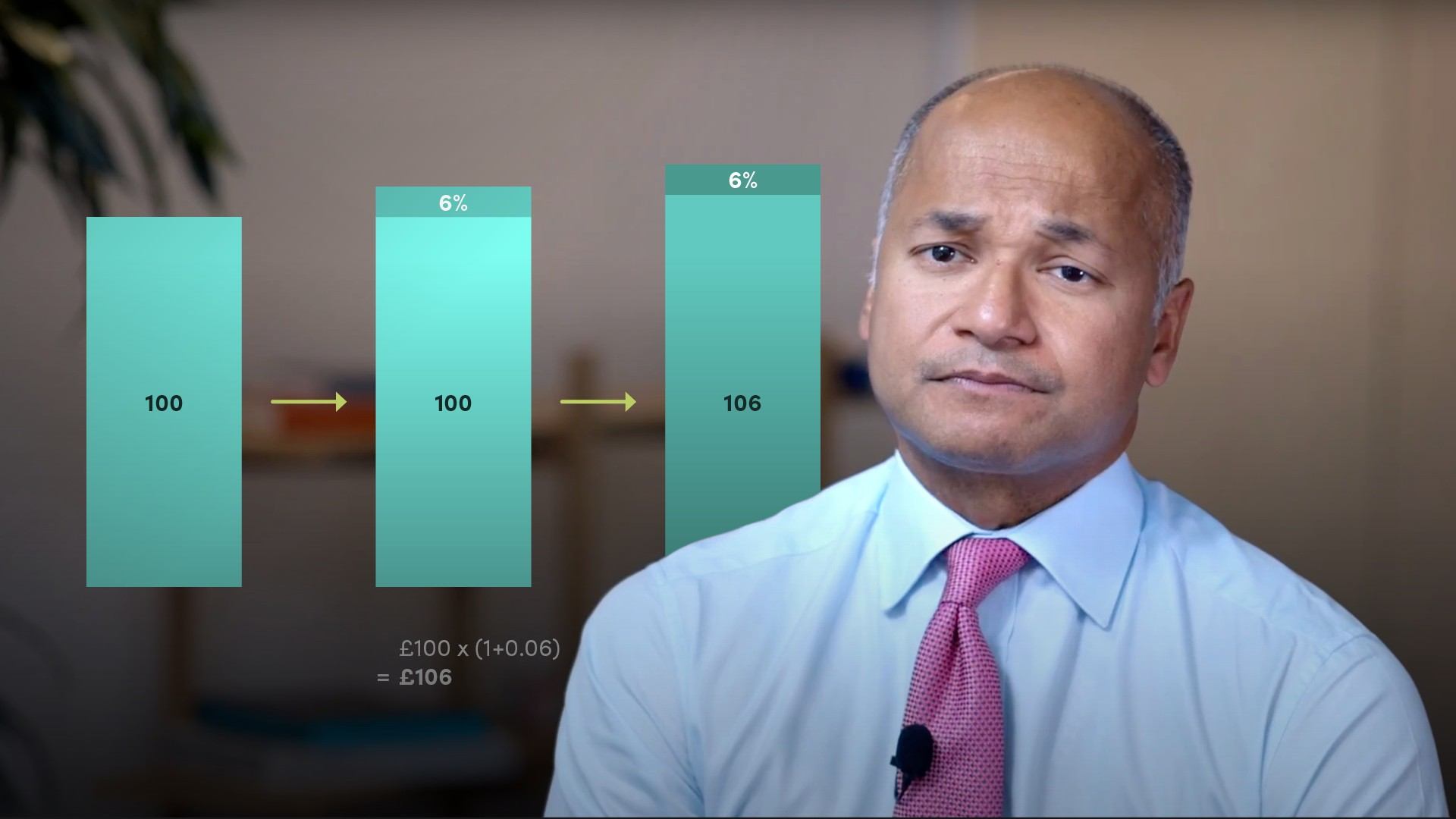09:4706:2605:2203:26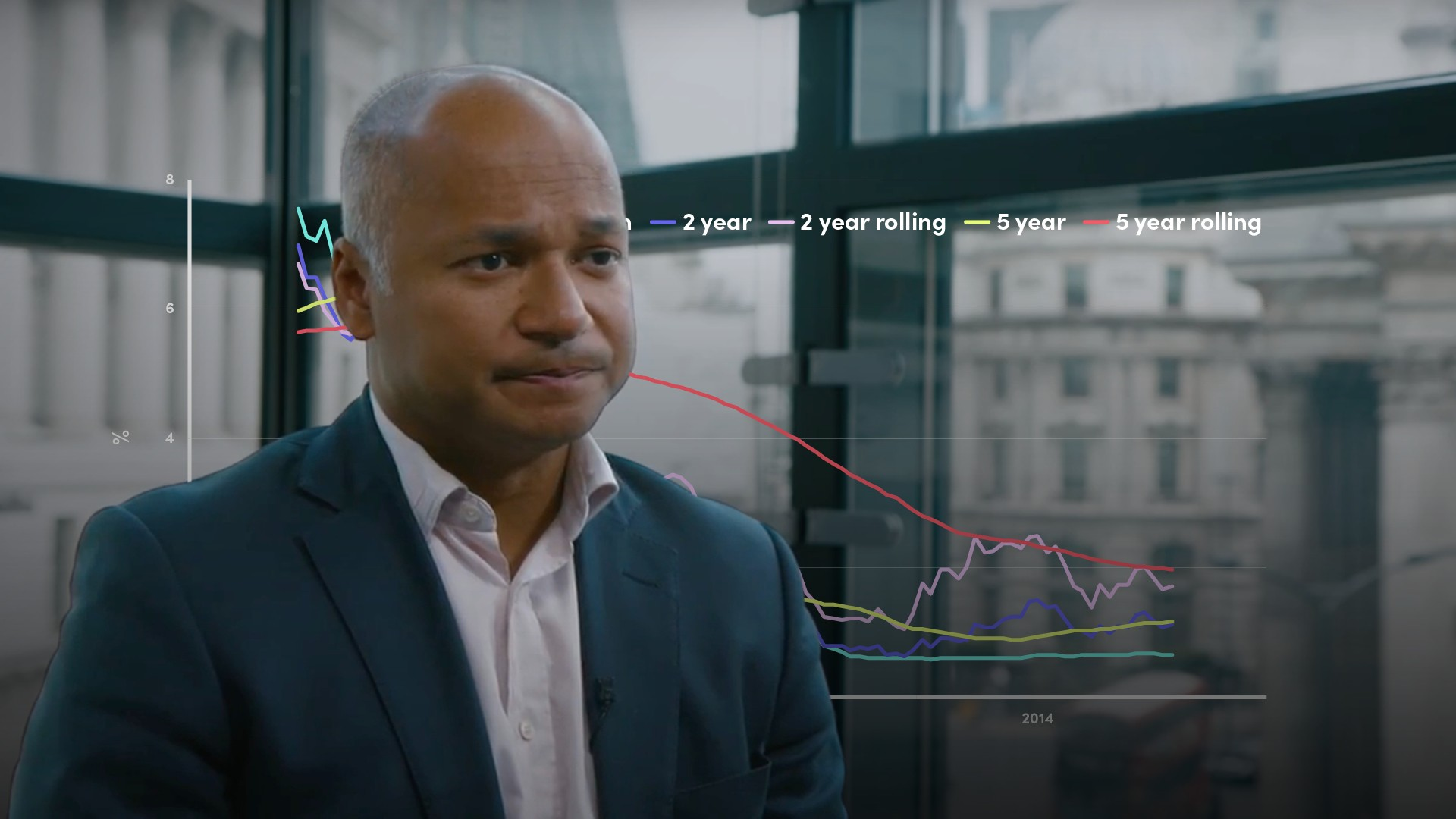14:5311:2807:3513:19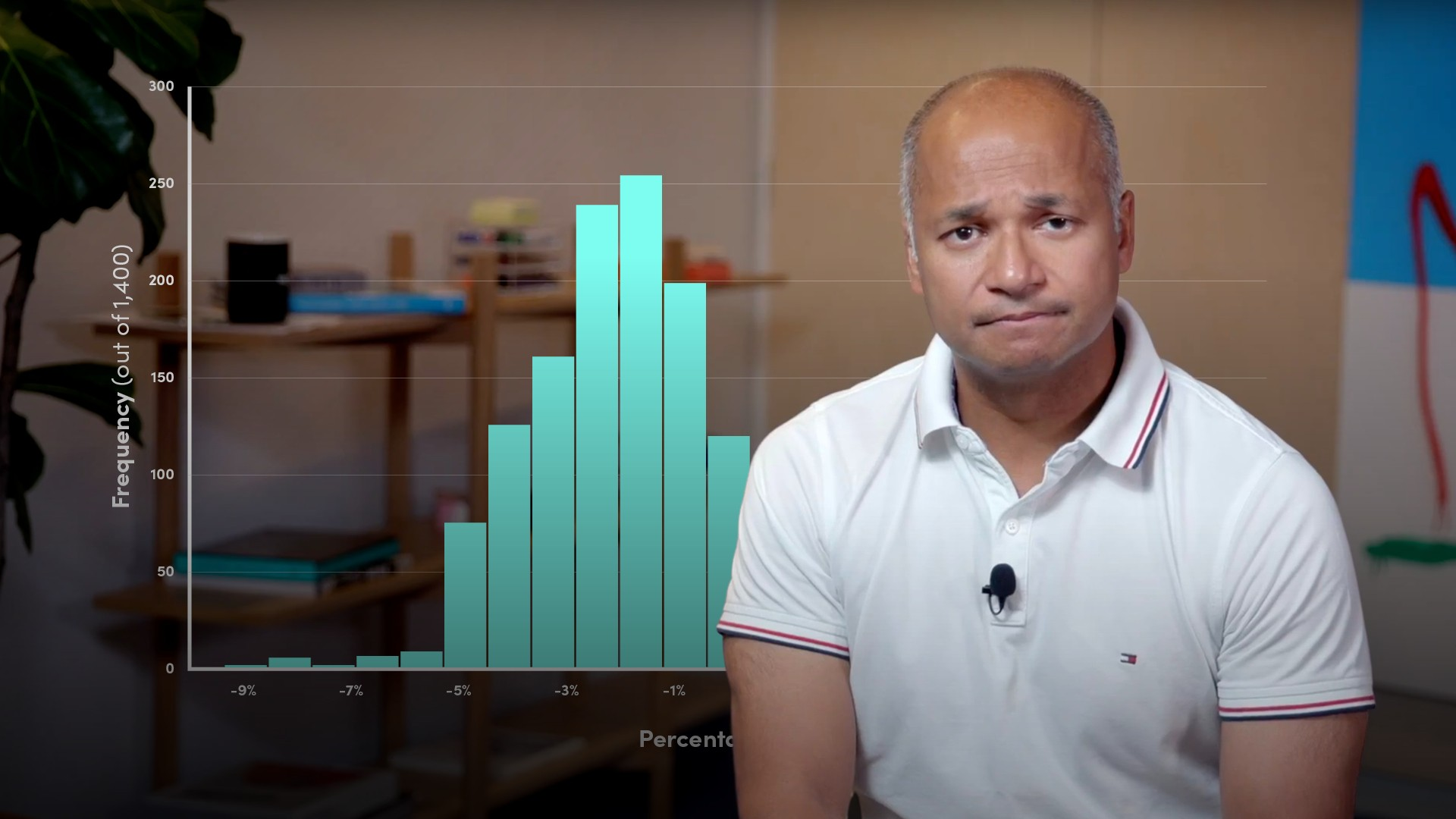15:25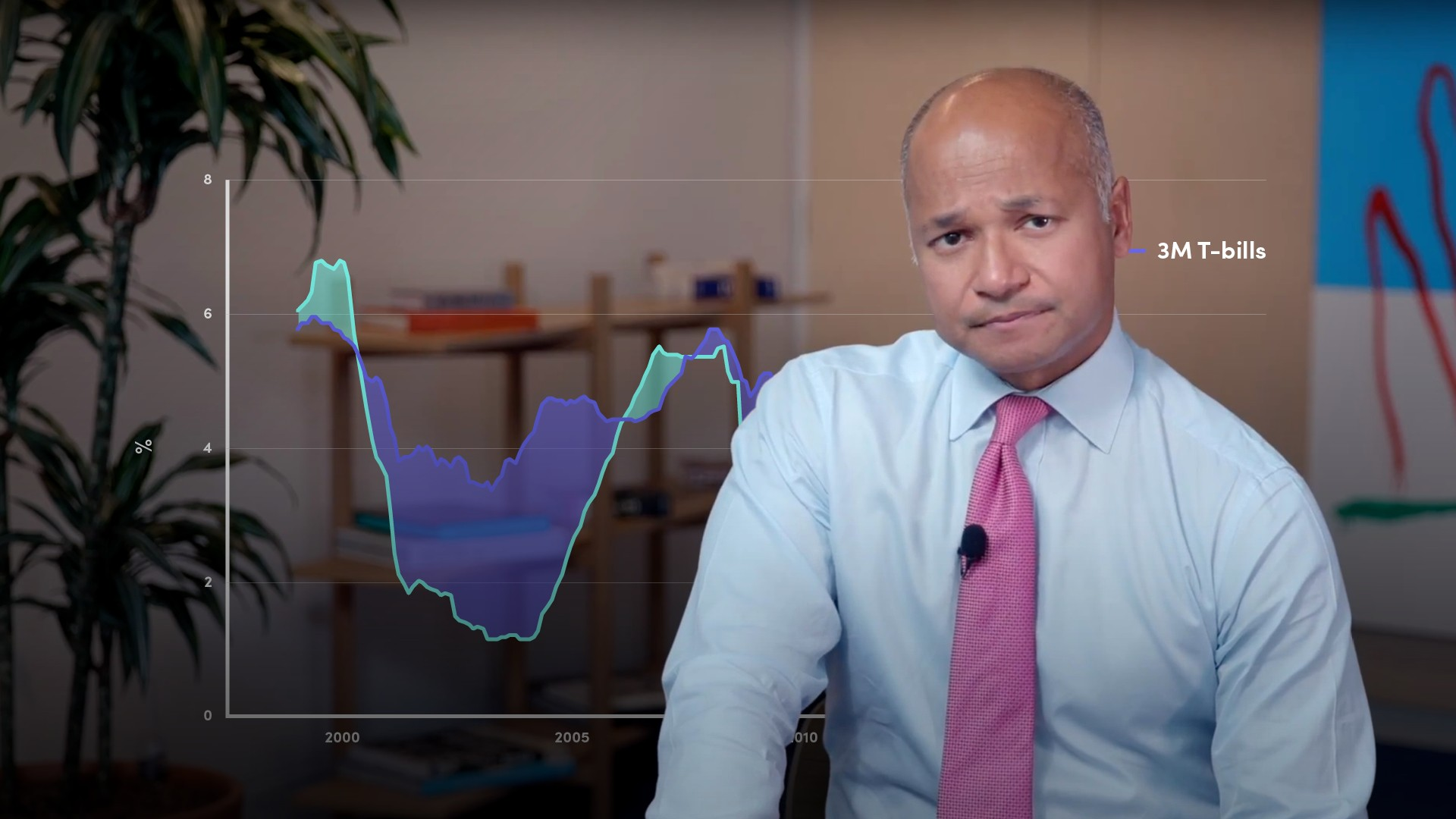11:47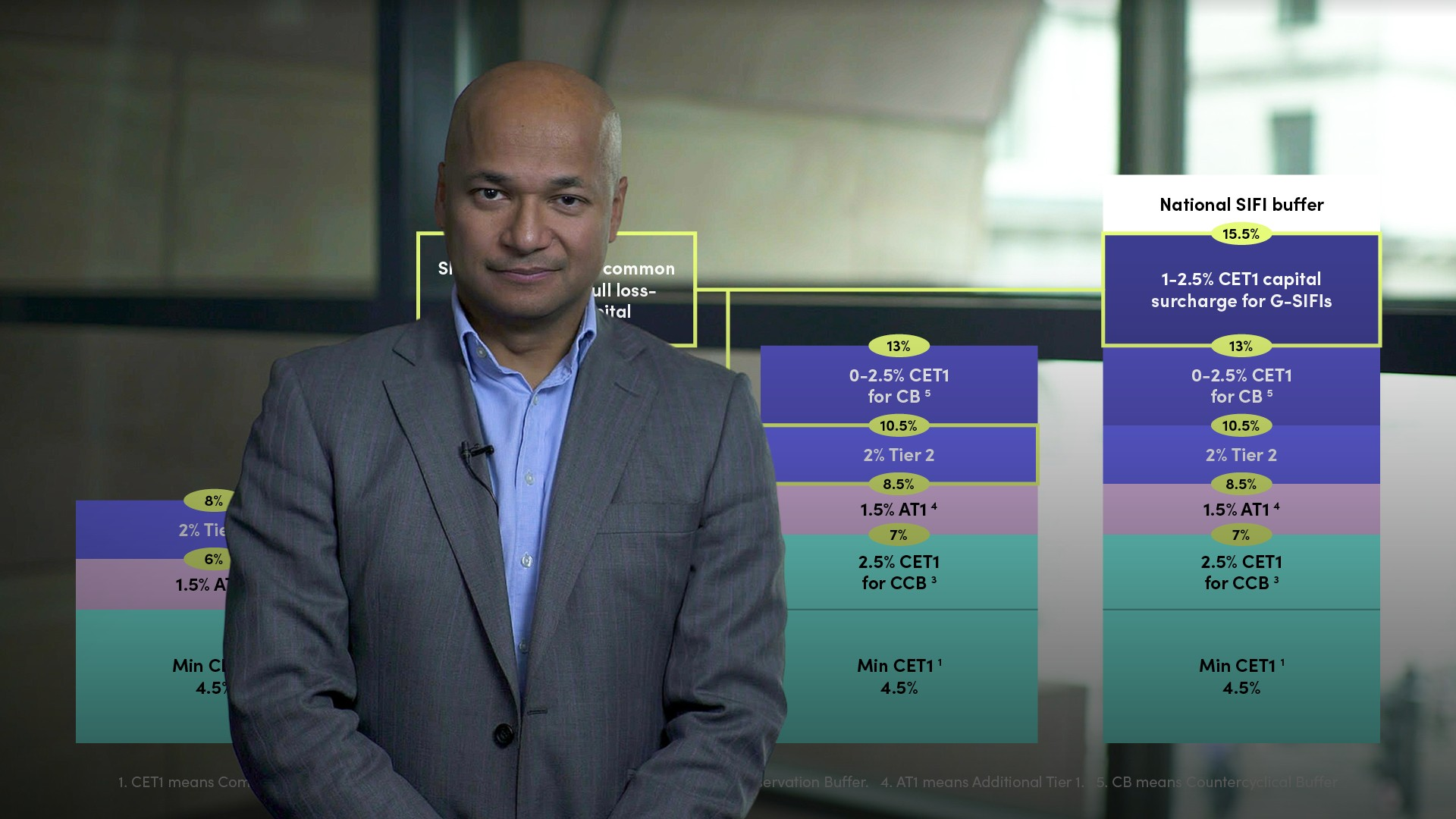11:0725:0919:44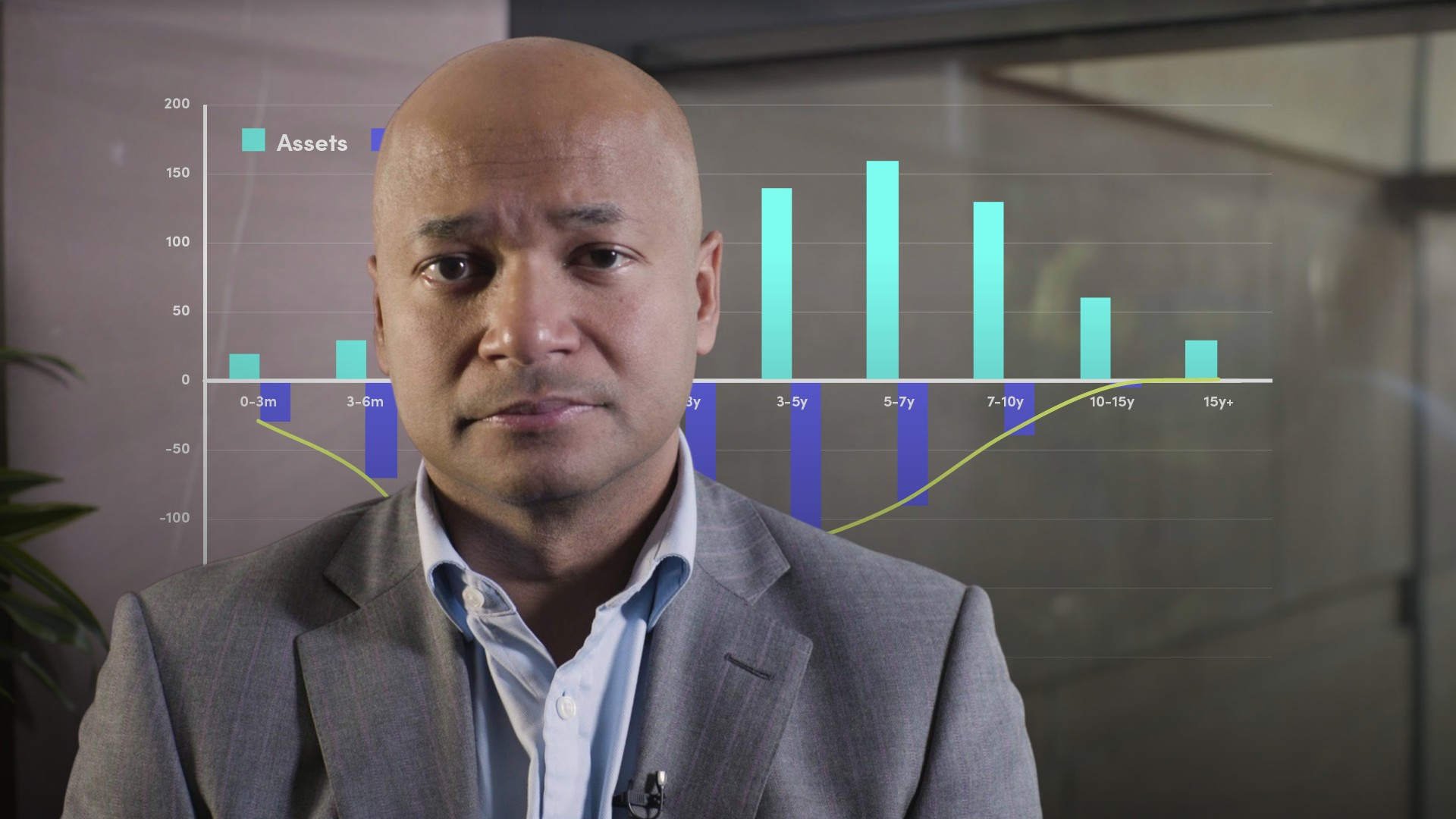17:0416:5910:01

## Related videosDerivatives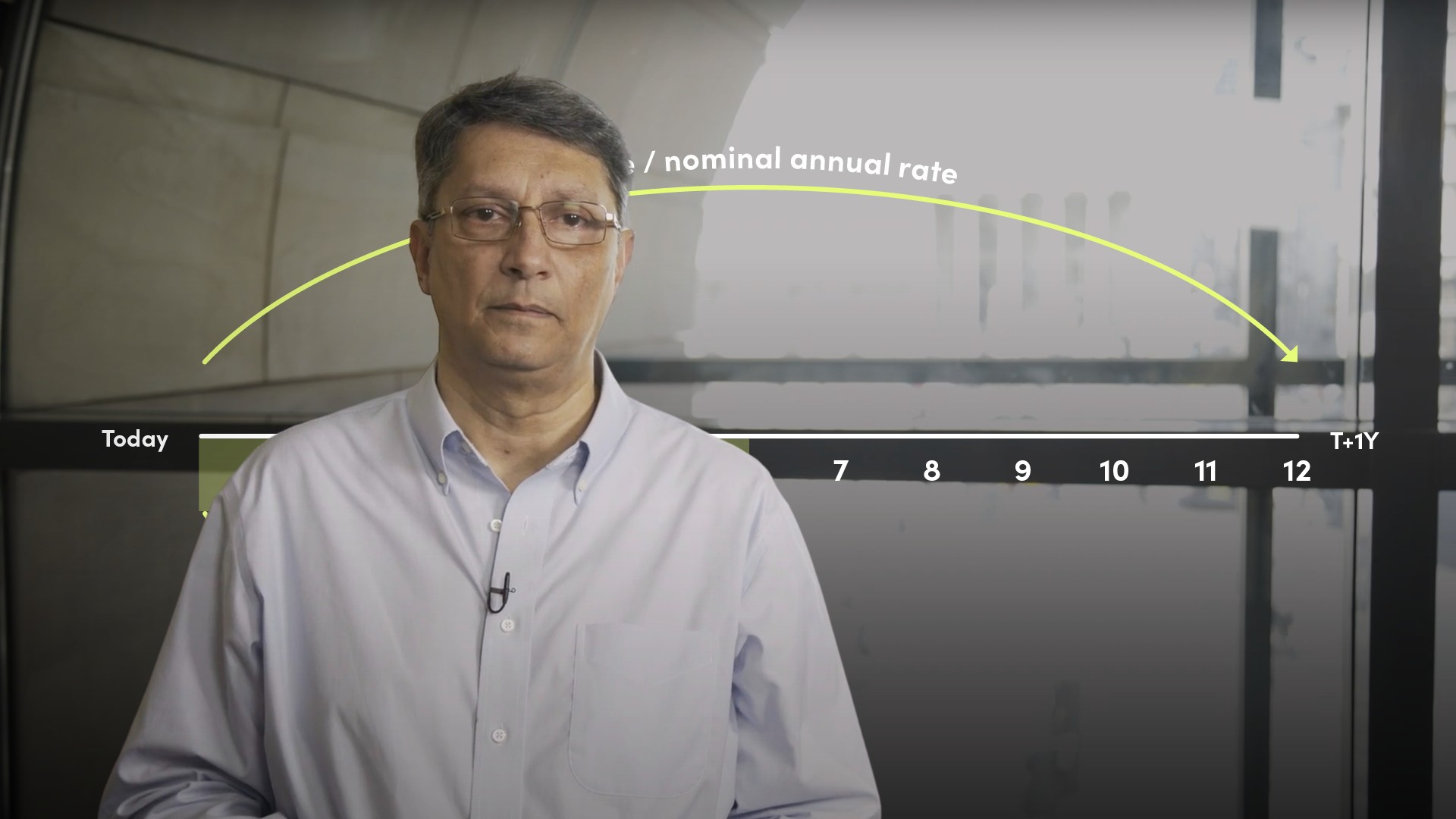Financial MathsFinancial MathsFinancial Maths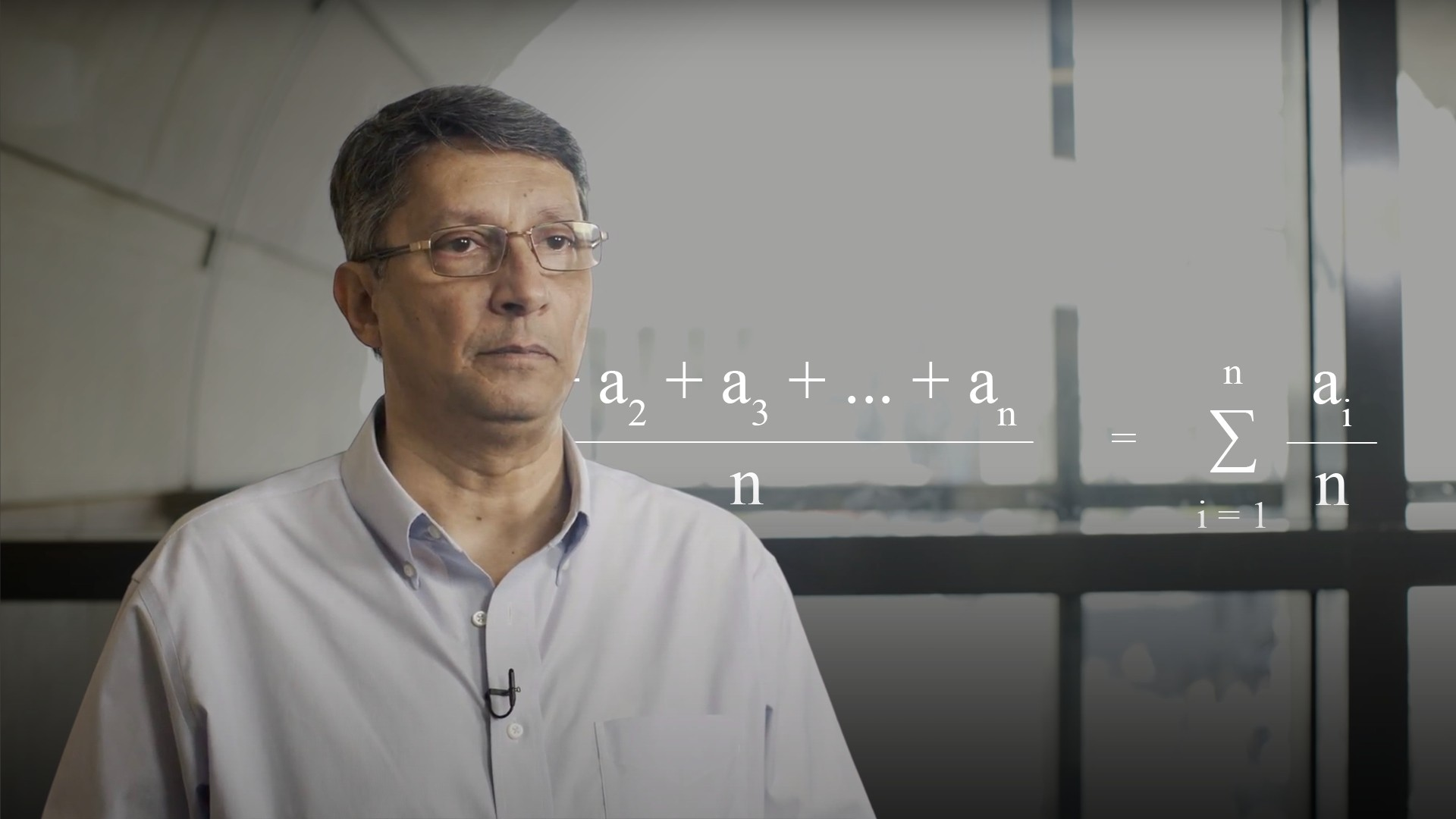Financial Maths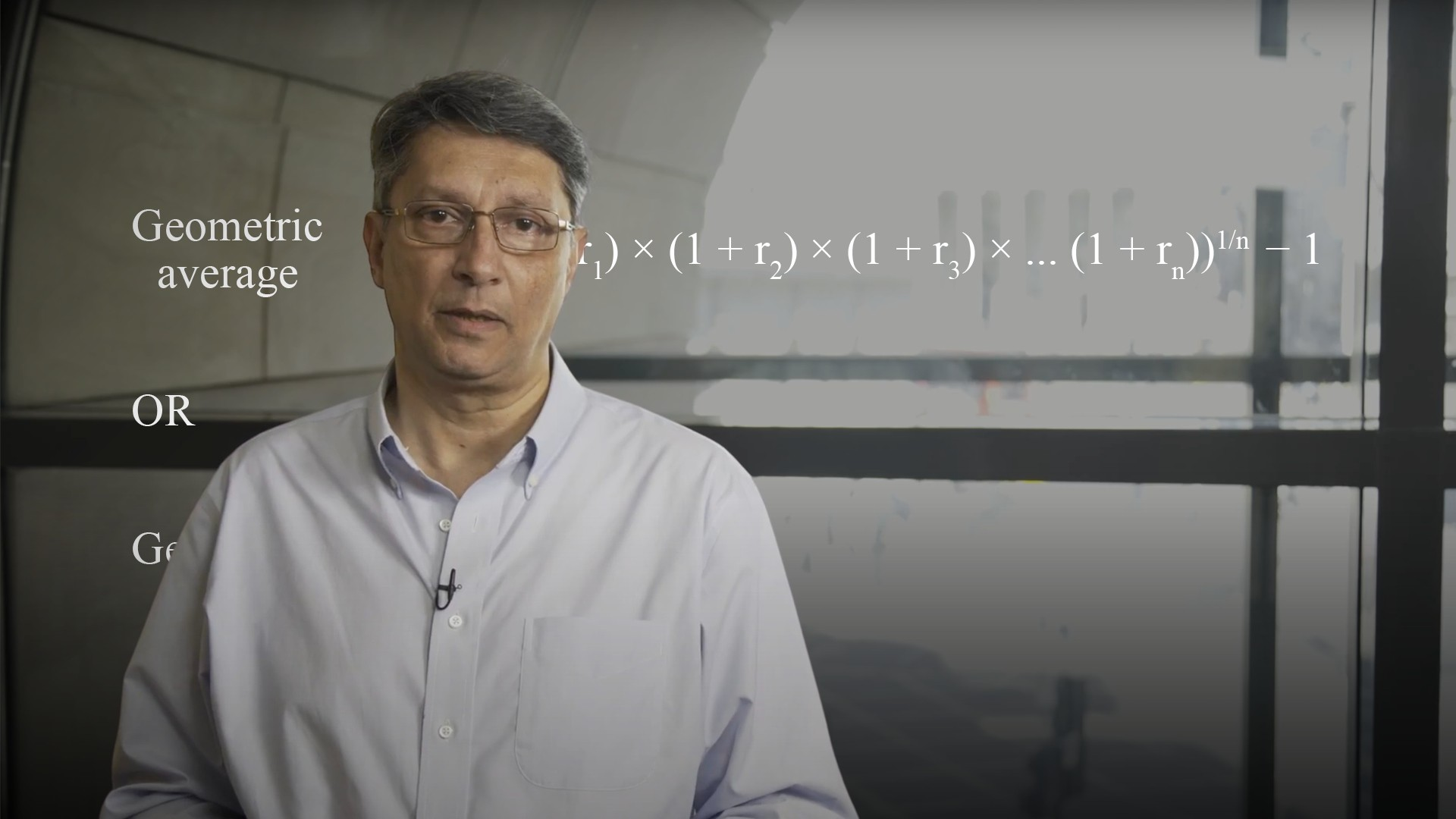Financial Maths### Accredited by